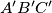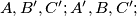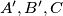### IMO Shortlist 1987 problem 12

Kvaliteta:
Avg: 0,0
Težina:
Avg: 0,0
Given a nonequilateral triangle$ABC$, the vertices listed counterclockwise, find the locus of the centroids of the equilateral triangles$A'B'C'$ (the vertices listed counterclockwise) for which the triples of points$A,B', C'; A',B, C';$ and$A',B', C$ are collinear.

Proposed by Poland.
Izvor: Međunarodna matematička olimpijada, shortlist 1987